# Optimal growth trajectories with finite carrying capacity

F. Caravelli, L. Sindoni, F. Caccioli, C. Ududec

Physical Review E 94, 22315 (2016)In presence of a finite carrying capacity, it is crucial to identify optimal strategies for maximal growth.

We consider the problem of finding optimal strategies that maximize the average growth rate of multiplicative stochastic processes. For a geometric Brownian motion, the problem is solved through the so-called Kelly criterion, according to which the optimal growth rate is achieved by investing a constant given fraction of resources at any step of the dynamics. We generalize these finding to the case of dynamical equations with finite carrying capacity, which can find applications in biology, mathematical ecology, and finance. We formulate the problem in terms of a stochastic process with multiplicative noise and a nonlinear drift term that is determined by the specific functional form of carrying capacity. We solve the stochastic equation for two classes of carrying capacity functions (power laws and logarithmic), and in both cases we compute the optimal trajectories of the control parameter. We further test the validity of our analytical results using numerical simulations.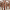#### Imaginary replica analysis of loopy regular random graphs

F. Lopez, T. Coolen

Sub. to Journal of Physics A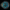#### Taming complexity

M. Reeves, S. Levin, T. Fink, A. Levina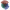#### The mathematical structure of innovation

T. Fink, I. Teimouri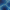#### Replica analysis of Bayesian data clustering

A. Mozeika,, A. Coolen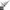#### Recursively divisible numbers

T. Fink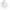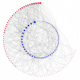#### Degree-correlations in a bursting dynamic network model

F. Vanni, P. Barucca

Journal of Economic Interaction and Coordination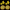#### Phase transition creates the geometry of the continuum from discrete space

R. Farr, T. Fink

Physical Review E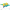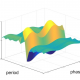#### Intelligently chosen interventions have potential to outperform the diode bridge in power conditioning

F. Liu, Y. Zhang, O. Dahlsten, F. Wang

Scientific Reports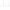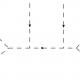#### Scale of non-locality for a system of n particles

S. Talaganis, I. Teimouri

Sub. to Physical Review D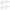#### How much can we influence the rate of innovation?

T. Fink, M. Reeves